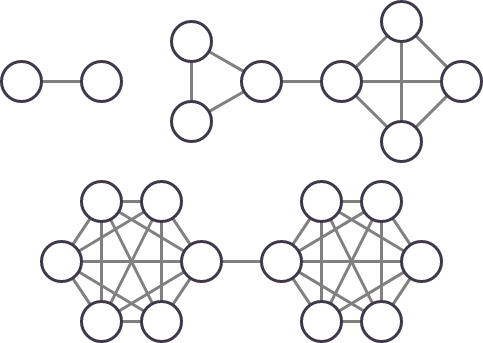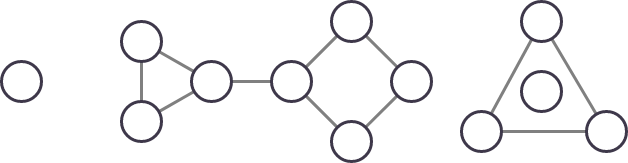## Dumbbell

View as PDF

Points: 1.20 (partial)
Time limit: 2.0s
Memory limit: 256M
Input: stdin
Output: stdout

Problem type
Allowed languages
C, C++, Java, Kotlin, Pascal, PyPy, Python, Scratch

An undirected graph is called a dumbbell if its vertices can be partitioned into ~2~ parts, such that:

• Each pair of vertices in the same part are connected by an edge.

• There exists exactly one edge that connects two vertices in different parts.

The following graphs are dumbbells:The following graphs are not dumbbells:The leftmost graph cannot be partitioned into two parts connected by an edge. In the middle graph, there is one part in which not all pairs of vertices are connected by an edge. The rightmost graph is not connected.

You are given an undirected graph with ~N~ vertices and ~M~ edges. The graph is simple: There is no self-loops, and there is no more than one edge between any pair of vertices. Calculate the least number of edges needed to add into the graph so that it becomes a dumbbell.

#### Input

The first line contains two integers ~n~ and ~m~ (~2 \le n \le 10^5~, ~0 \le m \le 10^5~), the number of vertices and edges of the given graph, respectively.

Each of the following ~m~ lines contain two integers ~u~ and ~v~ (~1 \le u, v \le n~, ~u \ne v~), denoting an edge between ~u~ and ~v~.

It is guaranteed that no two edges connect the same pair of vertices.

#### Output

Output a single integer, the least number of edges needed to add into the graph so that it becomes a dumbbell.

If there is no way to add edges to make the graph a dumbbell, output ~-1~.

#### Scoring

• Subtask 1 (~125~ points): ~n \le 500~

The total score for this problem is ~410~.

#### Sample Input 1

5 7
2 3
5 3
2 5
2 1
1 5
3 1
5 4


#### Sample Output 1

0


#### Sample Input 2

7 5
5 7
2 4
1 3
5 1
5 6


#### Sample Output 2

5


#### Sample Input 3

3 3
1 2
2 3
3 1


#### Sample Output 3

-1